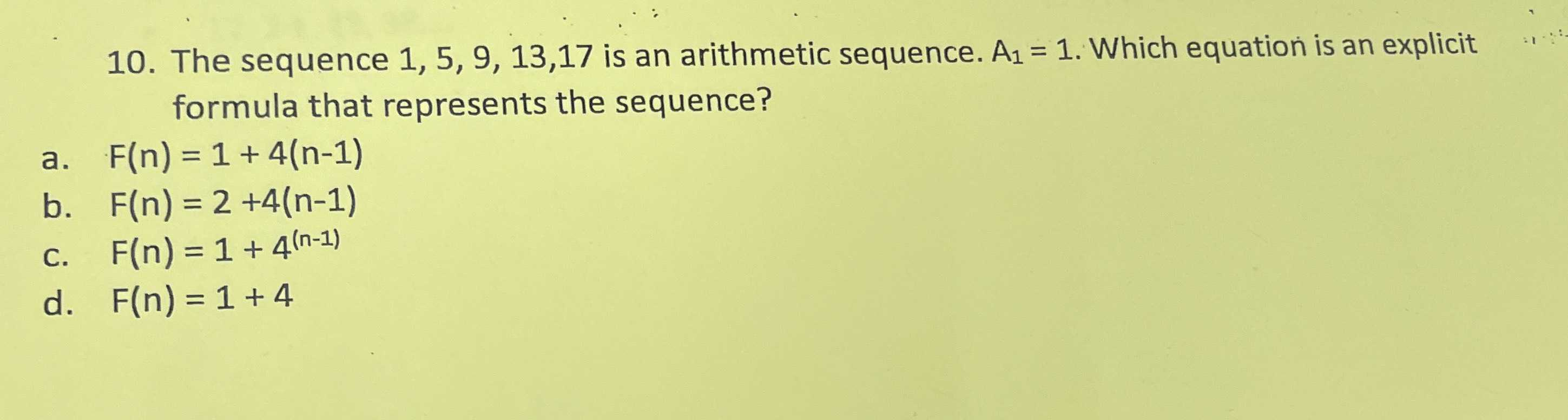### ¿Todavía tienes preguntas de matemáticas?

Pregunte a nuestros tutores expertos
Algebra
Pregunta10. The sequence $$1,5,9,13,17$$ is an arithmetic sequence. $$A _ { 1 } = 1$$ . Which equation is an explicit formula that represents the sequence?

a. $$F ( n ) = 1 + 4 ( n - 1 )$$

b. $$F ( n ) = 2 + 4 ( n - 1 )$$

c. $$F ( n ) = 1 + 4 ^ { ( n - 1 ) }$$

d. $$F ( n ) = 1 + 4$$